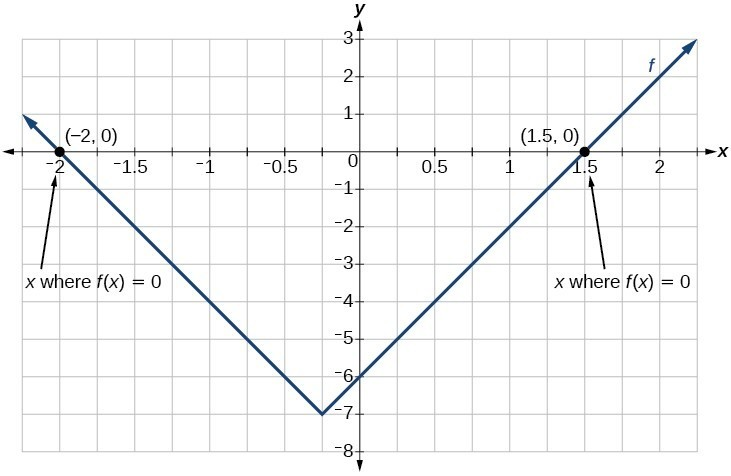## Solve an absolute value equation

Now that we can graph an absolute value function, we will learn how to solve an absolute value equation. To solve an equation such as ${8}=\left|{2}x - {6}\right|$, we notice that the absolute value will be equal to 8 if the quantity inside the absolute value is 8 or -8. This leads to two different equations we can solve independently.

$\begin{cases}2x - 6=8\hfill & \text{or}\hfill & 2x - 6=-8\hfill \\ 2x=14\hfill & \hfill & 2x=-2\hfill \\ x=7\hfill & \hfill & x=-1\hfill \end{cases}$

Knowing how to solve problems involving absolute value functions is useful. For example, we may need to identify numbers or points on a line that are at a specified distance from a given reference point.

An absolute value equation is an equation in which the unknown variable appears in absolute value bars. For example,

$\begin{cases}|x|=4,\hfill \\ |2x - 1|=3\hfill \\ |5x+2|-4=9\hfill \end{cases}$

### A General Note: Solutions to Absolute Value Equations

For real numbers $A$ and $B$, an equation of the form $|A|=B$, with $B\ge 0$, will have solutions when $A=B$ or $A=-B$. If $B<0$, the equation $|A|=B$ has no solution.

### How To: Given the formula for an absolute value function, find the horizontal intercepts of its graph.

1. Isolate the absolute value term.
2. Use $|A|=B$ to write $A=B$ or $\mathrm{-A}=B$, assuming $B>0$.
3. Solve for $x$.

### Example 4: Finding the Zeros of an Absolute Value Function

For the function $f\left(x\right)=|4x+1|-7$ , find the values of $x$ such that $\text{ }f\left(x\right)=0$ .

### Solution

$\begin{cases}0=|4x+1|-7\hfill & \hfill & \hfill & \hfill & \hfill & \hfill & \text{Substitute 0 for }f\left(x\right).\hfill \\ 7=|4x+1|\hfill & \hfill & \hfill & \hfill & \hfill & \hfill & \text{Isolate the absolute value on one side of the equation}.\hfill \\ \hfill & \hfill & \hfill & \hfill & \hfill & \hfill & \hfill \\ \hfill & \hfill & \hfill & \hfill & \hfill & \hfill & \hfill \\ \hfill & \hfill & \hfill & \hfill & \hfill & \hfill & \hfill \\ 7=4x+1\hfill & \text{or}\hfill & \hfill & \hfill & \hfill & -7=4x+1\hfill & \text{Break into two separate equations and solve}.\hfill \\ 6=4x\hfill & \hfill & \hfill & \hfill & \hfill & -8=4x\hfill & \hfill \\ \hfill & \hfill & \hfill & \hfill & \hfill & \hfill & \hfill \\ x=\frac{6}{4}=1.5\hfill & \hfill & \hfill & \hfill & \hfill & \text{ }x=\frac{-8}{4}=-2\hfill & \hfill \end{cases}$Figure 9

The function outputs 0 when $x=1.5$ or $x=-2$.

### Try It 4

For the function $f\left(x\right)=|2x - 1|-3$, find the values of $x$ such that $f\left(x\right)=0$.

Solution

Q & A

Should we always expect two answers when solving $|A|=B?$

No. We may find one, two, or even no answers. For example, there is no solution to $2+|3x - 5|=1$.

### How To: Given an absolute value equation, solve it.

1. Isolate the absolute value term.
2. Use $|A|=B$ to write $A=B$ or $A=\mathrm{-B}$.
3. Solve for $x$.

### Example 5: Solving an Absolute Value Equation

Solve $1=4|x - 2|+2$.

### Solution

Isolating the absolute value on one side of the equation gives the following.

$\begin{cases}1=4|x - 2|+2\hfill \\ -1=4|x - 2|\hfill \\ -\frac{1}{4}=|x - 2|\hfill \end{cases}$

The absolute value always returns a positive value, so it is impossible for the absolute value to equal a negative value. At this point, we notice that this equation has no solutions.

Q & A

In Example 5, if $f\left(x\right)=1$ and $g\left(x\right)=4|x - 2|+2$ were graphed on the same set of axes, would the graphs intersect?

No. The graphs of $f$ and $g$ would not intersect. This confirms, graphically, that the equation $1=4|x - 2|+2$ has no solution.

### Try It 5

Find where the graph of the function $f\left(x\right)=-|x+2|+3$ intersects the horizontal and vertical axes.

Solution